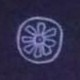# Entangling qubits

The rules of quantum mechanics have existed in their modern form since 1927 but  it was not until the 1980s that the possibility of quantum computing was first suggested. This was 40 years after the appearance of digital computers. Quantum computing is not an obvious idea if history is anything to go by.

Unlike the bits of digital computing, qubit states of a quantum computing (QC) can be rotated continuously (by probability-preserving unitary transformations). A typical quantum algorithm runs in three steps: (1) a register ofqubits is prepared in some known initial state (2) qubits are manipulated through a sequence of physical operations (corresponding to unitary transformations on multi-qubit states) (3) a probabilistic snapshot of the final quantum state is extracted (measurement). The algorithm runs in step (2). Step (3) is the read-out of results.

The list of elementary examples of QC is a short one. However, a quantum experiment often involves the same three steps above, and the result of the experiment always “computes” some quantity. Any quantum experiment has a QC interpretation.

Here I describe the quantum mechanics ofindistinguishable photons entering a beam-splitter. A pair of glass prisms glued together with a thin layer of resin form a device which partly reflects and partly transmits a beam of light. In classical optics a beam-splitter is described by complex transmission (,) and reflection (,) amplitudes that account for phase shifts of beams approaching from the left and right (unprimed and primed respectively). These amplitudes form a unitary matrixwhich has the general formIt turns out that all of the results discussed here are independent of phasesand. For definiteness chooseand. ThenIf the angle,. This is called “the standard 50:50 beamsplitter”. Here I allowto be different from.

The unitary matrixof classical optics carries over to the quantum case. Suppose that photons arrive at the beam splitter from orthogonal left or right channels as in Fig 1. The two orthogonal states are qubits denotedand. If a photo passes through the beam-splitter from the left, the photon state is rotated to. A photon in right channel is rotated toThe transition amplitudes areand. According to the rules of quantum mechanics the probability that a photon which entered from the left is detected in the left channel is the square modulus of the transition amplitude. The probability of finding the photon in right channel is. Ifthe probability is 50:50 as expected.Fig 1

Whenphotons are present, it is best to use many-photon Fock state notation.denotes a state withphotons entering in the left channel andphotons in the right channel (for example, the single photon stateabove would be represented by). In general the beam-splitter transforms an initial Fock stateinto an entangled Fock state. In general this is a superposition of allpossible states withphotons in left andright channels (satisfying) . Of course measurement (i.e. photon counting) will yield definite values,. In the language of QM, measurement is said to “collapse” the state. However, by repeating the experiment many times, the full probability distributioncan be found.

It is straightforward to calculate the general form of the beam-splitter transition amplitude(the quantum mechanical calculation takes the indistinguishable boson nature of photons into account. For details withsee reference  below). The answer is:

(1)Suppose that all photons enter in the same channel. In this case only a single term () survives in the sum in expression (1). The amplitudeis. The square modulus of this amplitude can easily be recognised as just the binomial distribution withplaying the role of probability.  In this case the beam-splitter is analogous to a classical analog computer, the Galton Board.Even though the computational details look very different, this is once again the expected result from classical probability.

What happens if photons enter in both left and right channels? Suppose that one photon enters each channel. Two terms survive in expression (1) but with opposite sign and the amplitude. This vanishes for a 50:50 beam-splitter. In other words the photons enter separately but always leave together. This effect, which appears to contradict intuition based on classical probability theory, is the experimentally observed Hong-Ou-Mandel effect in quantum optics. Generalising to the case wherephotons enter on the left and a single photon enters on the right, once again only two terms survive in expression (1)and, and the probability distribution is:where. This is very different from the binomial distribution because it is bimodal. For example, forand even, it vanishes whenwhich is where the binomial has its maximum. This is true even when.

What about the general case? The probability of observingphotons in the left channel given thatphotons enter the left channel () are shown below for a 200-qubit beamsplitter and:Another representation of the probability distribution () is shown below:

####The-photon beam-splitter experiment illustrates the preparation, transformation, measurement steps of QC and “computes” the square modulus of expression (1). Note that the factorials appearing in expression (1) are problematic on a classical computer whenwithout resorting to Stirling’s approximation.

Generalisations of the beam-splitter experiment to the multi-channel case known as Boson Sampling  have been proposed as a means to demonstrate quantum advantage, a computation that can be executed in QC but is infeasible on a classical computer. The quantity computed in Boson Sampling is closely related to the permanent of a matrix, a computationally hard problem on a classical computer.

0This site uses Akismet to reduce spam. Learn how your comment data is processed.